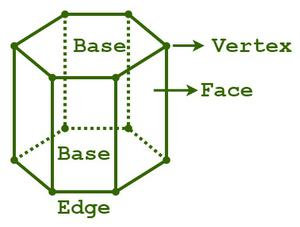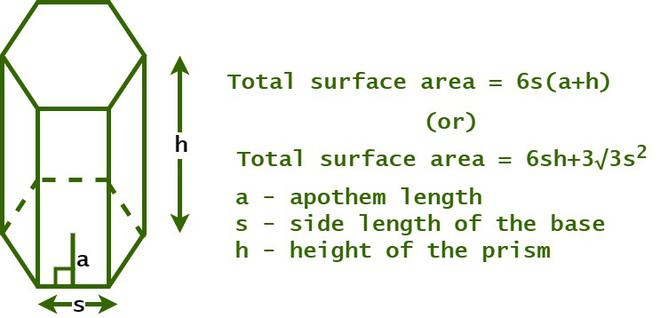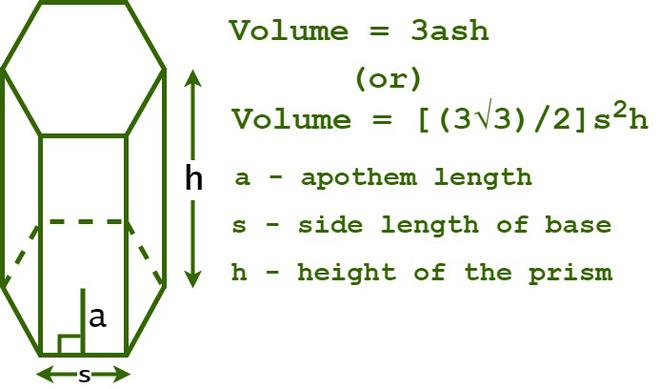Open in App
Not now

# Hexagonal Prism

• Last Updated : 24 Aug, 2022

A hexagonal prism is a three-dimensional geometric structure with two hexagonal bases connected by six rectangular faces. It is a polyhedron with eight faces, twelve vertices, and eighteen edges. It is also known as an octahedron as it has eight faces; two of the eight faces are hexagons, which are the bases of the prism, and the other six faces are rectangles, which are the lateral (or) side faces of the prism. The top and bottom faces of the hexagonal prism are in the shape of a hexagon and are congruent with each other.There are two kinds of hexagonal prisms, namely, a regular hexagonal prism and an irregular hexagonal prism.

• A regular hexagonal prism is a prism that has two hexagonal bases whose all sides are of the same length. In a regular hexagonal prism, the angles also measure the same.
• An irregular hexagonal prism is a prism that has two irregular hexagonal bases. All the sides of the base do not have the same length, and the measures of each angle are different.

## Surface Area of a Hexagonal Prism

The total area that is covered by the surfaces of a hexagonal prism is referred to as its surface area. The surface area of a prism is measured in terms of square units such as sq. m, sq. cm, sq. in, etc. A hexagonal prism has two types of areas just like other three-dimensional shapes: lateral surface area (LSA) and total surface area (TSA).

Let us consider a hexagonal prism that has an apothem length “a”, a base length “s”, and a height “h”. We know that the general formula to calculate the lateral surface area of a prism is the product of its base and height. So, the lateral surface area of the prism of a hexagonal prism is determined by calculating the product of the perimeter of the base of the hexagonal prism and its height.

The formula to determine the lateral surface area of the hexagonal prism is equal to the sum of the areas of its six rectangular faces. Thus,

Lateral surface area of hexagonal prism (LSA) = 6sh sq. units.

Where “s” is the length of the base edge, and
“h” is the height of the prism.The formula to determine the surface area of a hexagonal prism is given as follows:

Total Surface Area, TSA = 2×(Area of hexagonal base) + 6×(Area of rectangular faces) = 6s(a + h).

Total surface area of the hexagonal prism (TSA) = 6s(a + h) sq. units.

Where “a” is the apothem length,
“s” is the length of the base edge, and
“h” is the height of the prism.

The formula to determine the surface area of a hexagonal prism in the case of a regular hexagonal prism, TSA = 6sh + 3√3s2.

Total surface area of the hexagonal prism (TSA) = 6sh + 3√3s2 sq. units.

Where “s” is the length of the base edge, and
“h” is the height of the prism.

## Volume of a Hexagonal Prism

The volume of a hexagonal prism is the amount of space enclosed by it in three-dimensional space. It is also referred to as the amount of substance that it can hold, which is the capacity of a hexagonal prism. The formula for the volume of a hexagonal prism is equivalent to the product of its base area and height, which is measured in terms of cubic units such as cm3, m3, in3, etc.

The formula for finding the volume of a hexagonal prism is given as follows,

Volume of the Hexagonal Prism (V) = Base area × heightThe formula for calculating the volume of a hexagonal prism when the length of the edge of the base and height of the prism is known is given as follows.

Volume of the Hexagonal Prism (V) = [(3√3)/2]s2h

Where “s” is the length of the base edge, and
“h” is the height of the prism.

The formula for calculating the volume of a hexagonal prism when the apothem length, length of the edge of the base, and height of the prism are known is given as follows.

Volume of the Hexagonal Prism (V) = 3ash

Where “a” is the apothem length,
“s” is the length of the base edge, and
“h” is the height of the prism.

## Solved Example on Volume of a Hexagonal Prism

Example 1: Calculate the volume of a hexagonal prism with a base edge length of 15 cm and a height of 12 cm.

Solution:

Given data,

Length of the base edge (s) = 15 cm

The height of the prism (h) = 12 cm

We know that,

The volume of a hexagonal prism = [(3√3)/2]s2h

= (3/2) × (1.732) × (15)2 × 12

= 7,014.805 cu. cm

Hence, the volume of the hexagonal prism is 7,014.805 cu. cm.

Example 2: Determine the volume of the hexagonal prism if its height is 10 inches and its base area is given as 60 sq. in.

Solution:

Given data,

Base area = 60 sq. in

The height of the prism (h) = 10 inches

We know that

The volume of the hexagonal prism (V) = Base area × height

= 60 × 10 = 600 cu. in

Hence, the volume of the hexagonal prism is 600 cu. in.

Example 3: Calculate the volume of a hexagonal prism if its height is 13 cm, the length of each side of the base is 10 cm, and the apothem length is 8 cm.

Solution:

Given data,

Length of the base edge length (s) = 10 cm

Apothem length (a) = 8 cm

The height of the prism (h) = 13 cm

We know that

The volume of the hexagonal prism (V) = 3ash cubic units

= 3 × 8 × 10 × 13 = 3,120 cu. cm

Hence, the volume of the hexagonal prism is 3,120 cu. cm.

Example 4: Find the total surface area of a hexagonal prism if the length of each side of the base is 8 cm and the height is 10 cm.

Solution:

Given data,

Length of the base edge (s) = 8 cm

The height of the prism (h) = 10 cm

We know that,

The total surface area of the hexagonal prism = 6sh + 3√3s2

= 6 × 8 × 10 + 3√3 × (8)2

= 480 + 3√3 × 64

= 480 + 332.554 = 812.554 sq. cm

Thus, the total surface area of the hexagonal prism is 812.554 sq. cm.

Example 5: Determine the lateral surface area of a hexagonal prism with a base edge length of 12 cm and a height of 9 cm.

Solution:

Given data,

Length of the base edge length (s) = 12 cm

The height of the prism (h) = 9 cm

We know that,

The lateral surface area of a hexagonal prism = 6sh sq. units

LSA = 6 × 12 × 9

LSA = 648 sq. cm

Hence, the volume of the hexagonal prism is 648 sq. cm.

## FAQs on Hexagonal Prism

Question 1: What is the Volume of a Hexagonal Prism?

The volume of a hexagonal prism is the amount of space enclosed by it in three-dimensional space. It is also referred to as the amount of substance that it can hold, which is the capacity of a hexagonal prism. The formula for the volume of a hexagonal prism is equivalent to the product of its base area and height, which is measured in terms of cubic units such as cm3, m3, in3, etc.

The formula for finding the volume of a rectangular prism is given as follows,

The volume of the hexagonal prism (V) = Base area × height

Question 2: How to determine the Volume of a Hexagonal Prism?

Follow the steps given below to determine the volume of a hexagonal prism:

Step 1: Calculate the base area of the prism using the appropriate formula.
Step 2: Note down the value of the height of the given hexagonal prism.
Step 3: Substitute the values of the given dimensions in the formula, V = [(3√3)/2]s2h
Step 4: Finally, write the final value in appropriate cubic units.

Question 3: How can we determine the Volume of a Hexagonal Prism when its Base Area and Height are given?

We know that the general formula for calculating the volume of any prism is the product of its base area and its height. A hexagonal prism is a prism that has a hexagon as its base. So, we can also use the same formula for determining the volume of a hexagonal prism. As both the base area and the height of the hexagonal prism are given, the formula to determine the volume of a hexagonal prism is given as follows:

Volume of a hexagonal prism = Base Area × height.

Question 4: What will happen to the Volume of the Hexagonal Prism if its Height is reduced to Half?

We know that the formula for calculating the volume of the hexagonal prism is V = 3abh. Where “a” is the apothem length, “s” is the length of the base edge, and “h” is the height of the prism. So, the volume of the prism is directly proportional to its height. So, if the height is reduced to half, the new height will become (h/2). So, the volume of the new hexagonal prism will be 3ab (h/2) = (1/2) (3abh) = V/2. Therefore, we can conclude the volume will also be reduced by half.

Question 5: What is meant by the Surface Area of a Hexagonal Prism?

The total area that is covered by the surfaces of a hexagonal prism is referred to as its surface area. The surface area of a prism is measured in terms of square units such as sq. m, sq. cm, sq. in, etc. The formula to determine the surface area of a hexagonal prism is given as follows:

Total Surface Area, TSA = 2(Area of hexagon base) + 6(Area of rectangle faces) = 6s(a + h), where the “a” is apothem length of the prism, “s” is the length of the base edge, and “h” is the height of the prism.

The formula to determine the surface area of a hexagonal prism in the case of a regular hexagonal prism, TSA = 6sh + 3√3s2.

Question 6: How to determine the Lateral Surface Area of a Hexagonal Prism?